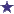# Days Supply Math Question: How Many Additional Tablets Are Needed?

by Wondering
(Boise, Idaho)Calculating Days Supply, And Figuring How Many Additional Tablets Are Needed

10 days supply: dosage 2 tablets 3x per day.

16 tablets per bottle.

How many additional tablets are needed?

### Comments for Days Supply Math Question: How Many Additional Tablets Are Needed?

Average RatingSep 02, 2017 Rating44 Additional Tablets Are Needed to Fill this Rx by: David To start with, lets first figure out how many total tablets are needed for the 10 days, and then, second, we can answer the question by subtracting the tablets "on hand" with the total needed, and then we will have our answer. So let's get started. We want to figure out how many total tablets are being taken each day. Once we know that, we can multiply that number by 10 since we want a 10-day-supply. The question states that the sig calls for 2 tablets, 3 times daily. 2 X 3 = 6 tablets per day. Next, we multiply 6 by 10 (days) which equals #60 tablets are needed. Finally, we subtract 16 ("On Hand") from 60 which equals 44. So we need an additional 44 tablets to fill the entirety of this prescription.